Bihar Board 12th Maths Important Questions Long Answer Type Part 3

BSEB Bihar Board 12th Maths Important Questions Long Answer Type Part 3 are the best resource for students which helps in revision.

Bihar Board 12th Maths Important Questions Long Answer Type Part 3

Continuity and Differentiability

Question 1.
Find the relation b/w a & b so that the function (x) defined by
$$f(x)=\left\{\begin{array}{ll} a x+b & \text { if } x \leq 3 \\ b x+3 & \text { if } x>3 \end{array}\right.$$ is continuous at x = 3
Solution: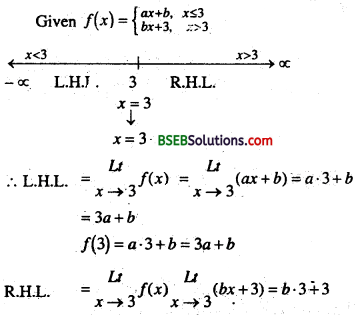= 3a + b
f(3) = a.3 + 8 = 3a + 8R.H.L. = $$\begin{array}{c} L t \\ x \rightarrow 3 \end{array}$$f(x) $$\begin{array}{c} L t \\ x \rightarrow 3 \end{array}$$(b x+3)=b.3 + 3
= 3b + 3
∵ f(x) is continuous at x = 3.
∴ LHX =RHL= f(3)
∵ 3a + b = 3b + 3′
⇒ 3a + b – 3b = 3
⇒ 3a – 2b = 3

Question 2.
Find k if f(x) = $$f(x)=\left\{\begin{array}{cc} \frac{k \cos x}{\pi-2 x} & \text { if } x \neq \frac{\pi}{2} \\ 3 & \text { if } x=\frac{\pi}{2} \end{array}\right.$$ is continuous at x = $$\frac{\pi}{2}$$
Solution:
Given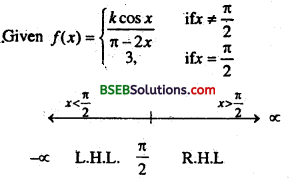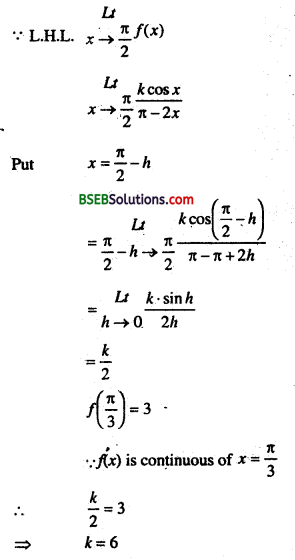Question 3.
Find $$\frac{d y}{d x}$$
(i) y = (cos x . cos 2x) . cos 3x
(ii) x = 2at2 y = at4
(iii) x = a cosθ, y = bsecθ
(iv) x = 4t, y = $$\frac{4}{t}$$
(v) x = cos θ – cos2θ, y = sinθ – sin2θ
(vi) x = a(cost + log tan $$\frac{t}{2}$$), y = a sin t
Solution:
(i) ∵ y = (cos x . cos 2x) . cos 3x
Diff w.r. to x
$$\frac{d y}{d x}$$ = (cosx . cos2x) . d.c. of cos 3x + cos 3x . d.c. of (cos x . cos 2x)
or, $$\frac{d y}{d x}$$ = – cosx . cos 2x . sin 3x . 3 + cos 3x . (cos x d.c. of cos 2x + cos 2x d.c. of cos x)
or, $$\frac{d y}{d x}$$ = – 3cos x . cos2x . sin3x + cos3x . (-cos x . sin2x – cos2x.sinx)
or, $$\frac{d y}{d x}$$ = -3cosx . cos2x sin3x – cos3x . sin(2x + x)
∴ $$\frac{d y}{d x}$$ = -3cosx . cos2x sin3x – sin3x cos3x

(ii) x = 2at2,
Diff w.r. to t
$$\frac{d x}{d t}$$ = 4at …………..(i)

y = at4
Diff w.r. to t
$$\frac{d y}{d t}$$ = 3at3 ………… (ii)

Eqn. (ii) ÷ Eqn. (i)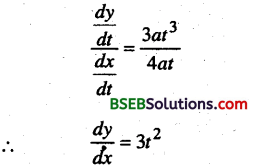(iii) x = a cos θ
Diff w.r. to θ
$$\frac{d x}{d t}$$ = -asin θ……………. (i)

y = b sec θ
Diff w.r. to θ
$$\frac{d y}{d t}$$ = b secθ . tan θ……………. (ii)

Eqn. (ii) ÷ Eqn. (i)
$$\frac{d y}{d x}=\frac{b \sec \theta \cdot \tan \theta}{-a \sin \theta}$$

(iv) x = 4t
Diff w.r. to t
$$\frac{d x}{d t}$$ = 4 …………… (i)

y = $$\frac{4}{t}$$
Diff w.r. to t
$$\frac{d y}{d t}=-\frac{4}{t^{2}}$$ ……………….. (ii)

Eqn. (ii) ÷ Eqn. (i)(v) x = cos θ – cos 2 θ
Diff. w.r. to θ
∴ $$\frac{d x}{d \theta}$$ = -sinθ + sin 2θ.2 ……….(i)

& y = sinθ – sin 2θ
Diff. w.r. to θ
∴ $$\frac{d y}{d \theta}$$ = cosθ – cos2θ.2 ……….(ii)
Eqn. (ii) ÷ Eqn. (i)(vi) x = a(cos t + log tan $$\frac{t}{2}$$), y = a sin t
Diff w.r.to tQuestion 4.
Show that f(x) = $$\left\{\begin{array}{l} 12 x-13, x \leq 3 \\ 2 x^{2}+5, x>3 \end{array} x=3\right.$$ is differentiable at x = 3. Also find f'(3).
Solution:∵ L.H.S = R.H.S at x = 3
∴ f(x) is differentiable at x = 3 and f'(3) = 12.Question 5.
For whal choice of a & b is the function :
$$f(x)=\left\{\begin{array}{c} x^{2}, x \leq c \\ a x+b, x>c \end{array}\right.$$ is differentiable at x = c.
Solution:
It is given that f(x) is differentiable at x = c
∴ f(x) will be continuous of x = c.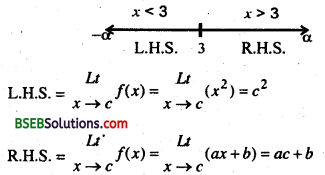f(c) = c2
∵ L.H.S. = R.H.S.=/(c)
∴ c2 = ac + b = c2
Now f(x) is differentiable at x = c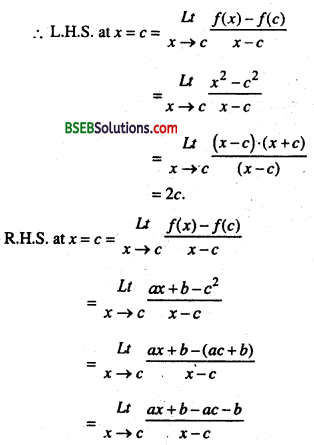= $$\underset{x \rightarrow c}{Lt}$$ $$\frac{a(x-c)}{(x-c)}$$= a
∴ L.H.S. = R.H.S.
∴ a = 2c …(ii)
from eqn. (i) & eqn. (ii)
c2 = 2c2 + b
=> b = c2 & a = 2c

Question 6.
IF f(x) = $$\left\{\begin{array}{c} x^{2}+3 x+a, x \leq 1 \\ b x+2, \quad x>1 \end{array}\right.$$ is every where differentiable. Find the values of a & b.
Solution:
For x ≤ 1, f(x) = x2 + 3x + a = polynomial
For x > 1,
f(x) = bx + 2 = polynomial,
∵ Polynomial function is every where differentiable.
∴ Therefore f(x) is differentiable for all x > 1 and also for all x < 1.
∵ f(x) is continuous at x = 1
∴ L.H.S. = R.H.S. = f(1)
or $$\begin{array}{c} L t \\ x \rightarrow 1 \end{array}$$ (x2 + 3x + a) = $$\begin{array}{c} L t \\ x \rightarrow 1 \end{array}$$(bx + 2) = 12 + 3.1 + a
or, 12 + 3.1 + a = b.1 + 2 = 12 + 3.1 + a
or, 4 + a = b + 2
⇒ a – b + 2 = 0 ….(i)
Again f(x) is differentiable at x = 1
∴ L.H.S. at x = 1 = R.H.S. at x = 1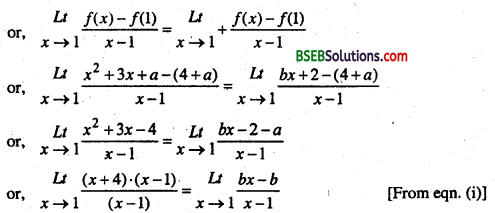or, 5 = b ⇒ B = 5
From eqn. (i),
a – 5 + 2 = 0
or, a – 3 = 0
∴ a = 3Differentiation

Question 1.
Differentiate the following functions w.r. to x from 1st principles.
(i) sin$$\sqrt{x^{2}+a x+1}$$
(ii) $$\sqrt{e^{\tan x}}$$
Solution: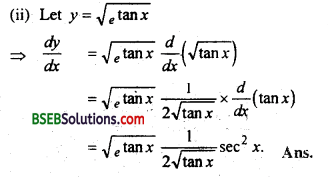Question 2.
If y = $$\left[x+\sqrt{x^{2}+a^{2}}\right]^{n}$$ then prove that $$\frac{d y}{d x}=\frac{n y}{\sqrt{x^{2}+a^{2}}}$$
Solution:
Let y = $$\left[x+\sqrt{x^{2}+a^{2}}\right]^{n}$$
Diff w.r.to x.Question 3.
If y = $$\sqrt{\frac{1-x}{1+x}}$$ then prove that (1 – x2)$$\frac{d y}{d x}$$ + y = 0
Solution:
Given y = $$\sqrt{\frac{1-x}{1+x}}$$
Diff w.r.to x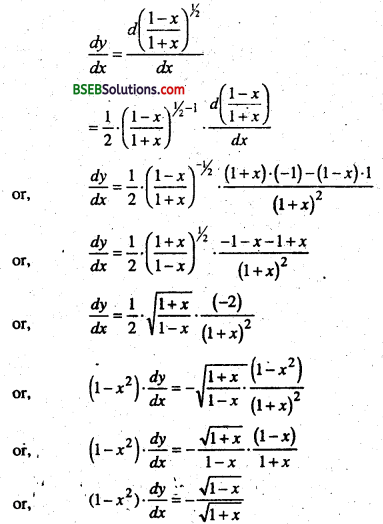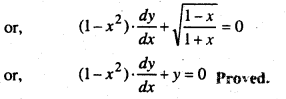Question 4.
Prove that $$d\left\{\frac{x \cdot \sqrt{a^{2}-x^{2}}+\frac{a^{2}}{2} \cdot \sin ^{-1} x}{d x}=\sqrt{a^{2}-x^{2}}\right.$$
Solution:Question 5.
If xy = ex-y prove that $$\frac{d y}{d x}=\frac{\log x}{\left(1+\log x^{2}\right.}$$
Solution:
Given xy = ex-y
or eylogx = ex-y
ylogx = x – y
ylog x +y = x
or y(1 + log x) = x
or y = $$\frac{x}{1+\log x}$$
Diff. w.r.to xQuestion 6.
If (cosx)y = (sin y)x, find $$\frac{d y}{d x}$$
Solution:
Given (cos x)2 = (sin y)x
or, log (cos x)y = log(sin y)x
or, y. log (cos x) = x log (siny)
Diff . w.r.to x
y.$$\frac{1}{\cos x}$$. (-sinx) + log(cos x)$$\frac{d y}{d x}$$ = x.$$\frac{1}{\sin y}$$ . cos y. $$\frac{d y}{d x}$$ + log(sin y)
or, -y tan x + log(cosx)$$\frac{d y}{d x}$$ x cot y. $$\frac{d y}{d x}$$ + log(sin y)
or $$\frac{d y}{d x}$$. [log(cos x) – xcot y] = x cot y] = log(sin y) + y tan x
∴ $$\frac{d y}{d x}=\frac{\log (\sin y)+y \tan x}{\log (\cos x)-x \cot y}$$

Question 7.
Differentiate sin-1($$\frac{2 x}{1+x^{2}}$$) w. r. to tan-1 x, -1 < x < 1
Solution:
Let u = sin-1($$\frac{2 x}{1+x^{2}}$$)
or u = 2tan-1 x and v = tan-1 x&
Diff . w.r.to x
∴ $$\frac{d u}{d x}=2 \cdot \frac{1}{1+x^{2}}$$ ……………… (i)
$$\frac{d v}{d x}=\frac{1}{1+x^{2}}$$ …………………… (ii)
Eqn(i) / Eqn(ii)
$$\frac{d u}{d v}$$ = 2

Question 8.
If y = log$$\sqrt{\tan x}$$ then $$\frac{d y}{d x}$$ = ?
Solution:
Given y = log$$\sqrt{\tan x}$$ then $$\frac{d y}{d x}$$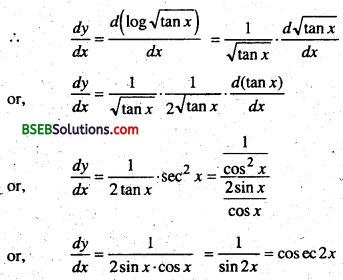Question 9.
If y = sin{tan(log x)}, find $$\frac{d y}{d x}$$
Solution:
∵ y = sin{tan(log x)}
⇒ $$\frac{d y}{d x}=\frac{d}{d x}$$ [sin{tan(log x)}
= cos{tan(logx)) x $$\frac{d}{d x}$${tan(logx)}
= cos{tan(logx)) x sec2(logx) x $$\frac{d}{d x}$$ {log x}
= cos{tan(Iog x)) x sec2(log x) x $$\frac{1}{x}$$
= $$\frac{1}{x}$$sec2(logx)cos{tan(logx)).

Question 10.
Integrate $$\int_{0}^{\pi / 4} \cos ^{2} x d x$$
Solution: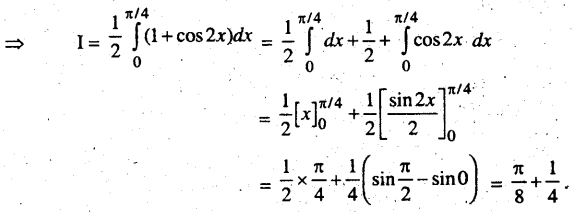Question 11.
If y = sinx2, find $$\frac{d y}{d x}$$
Solution:
∵ y = sinx2
Diff w.r. to x we get
∴ $$\frac{d y}{d x}=\frac{d}{d x}$$ (sin x2) = cos x2 $$\frac{d}{d x}$$ (x2) = 2cos x2.

Question 12.
If y = tan3 θ find $$\frac{d y}{d \theta}$$
Solution:
y = tan3 θ
Diff w.r. to θ we get
$$\frac{d y}{d \theta}=\frac{d}{d \theta}$$ (tan3 θ) = 3tan3 θ $$\frac{d}{d \theta}$$ (tan θ) = 3 tan2θ sec2θ

Question 13.
If y = cot-1 $$\frac{1-x}{1+x}$$ then prove that $$\frac{d y}{d x}=\frac{1}{1+x^{2}}$$
Solution:
∵ y = cot-1 $$\frac{1-x}{1+x}$$
put x = tan θ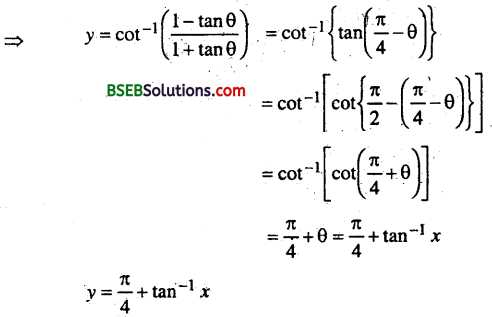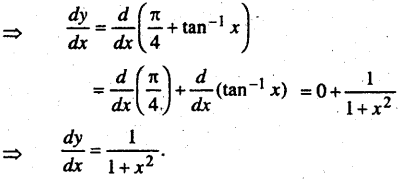Question 14.
If y = $$\tan ^{-1} \sqrt{\frac{1+\sin x}{1-\sin x}}$$ then find the value of $$\frac{d y}{d x}$$
Solution:
y = $$y=\tan ^{-1} \sqrt{\frac{1+\sin x}{1-\sin x}}$$